# RS Aggarwal Solutions for Class 6 Chapter 13 Angles and their Measurement Exercise 13C

This exercise mainly consists of problems which help in constructing angles using a protractor and step followed to construct a perpendicular line. We know that protractor is an instrument which is used for measuring or constructing an angle of a given measure. RS Aggarwal Solutions are designed by experts based on the latest CBSE textbook. To know more about the concepts, RS Aggarwal Solutions for Class 6 Chapter 13 Angles and their Measurement Exercise 13C PDF can be downloaded for free.

## Download PDF of RS Aggarwal Solutions for Class 6 Chapter 13 Angles and their Measurement Exercise 13C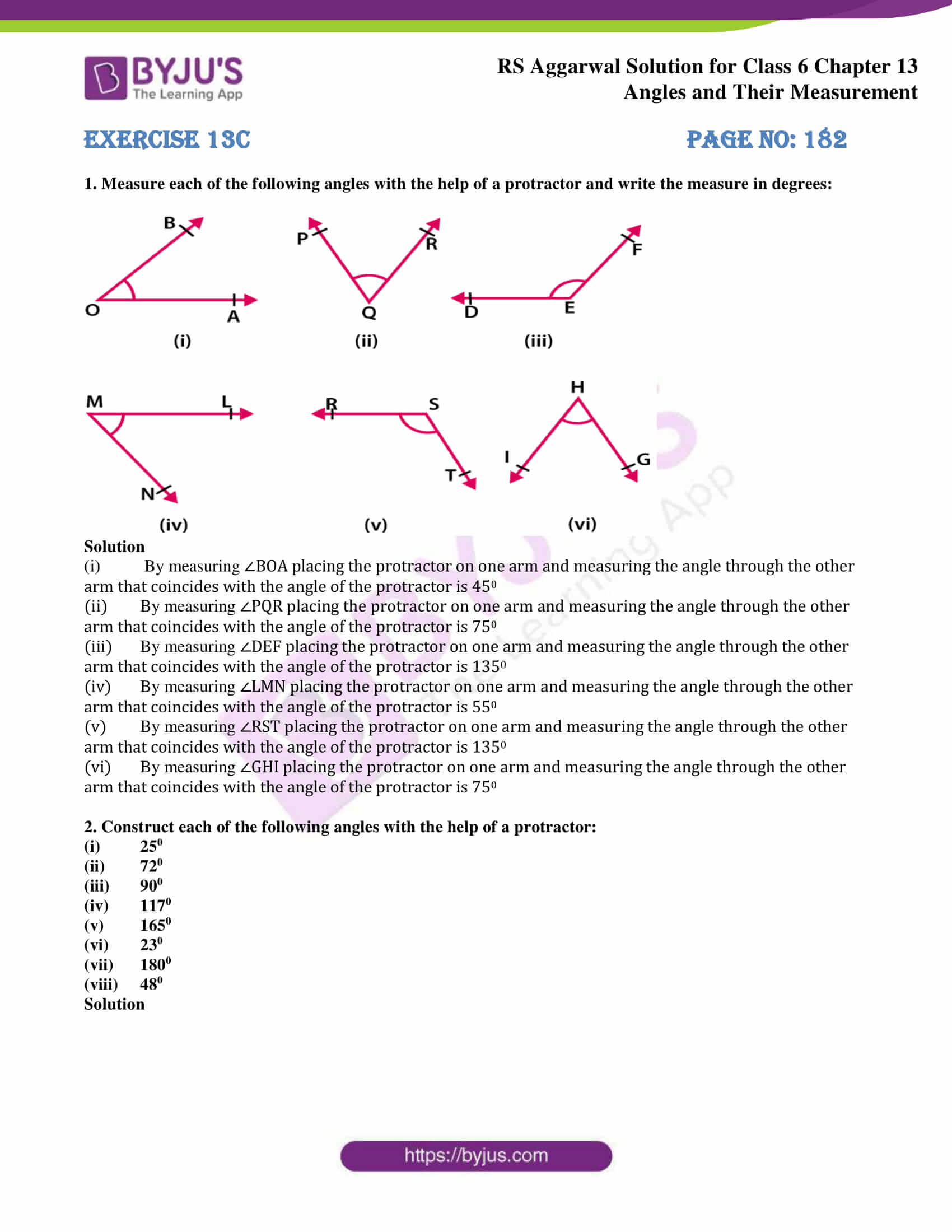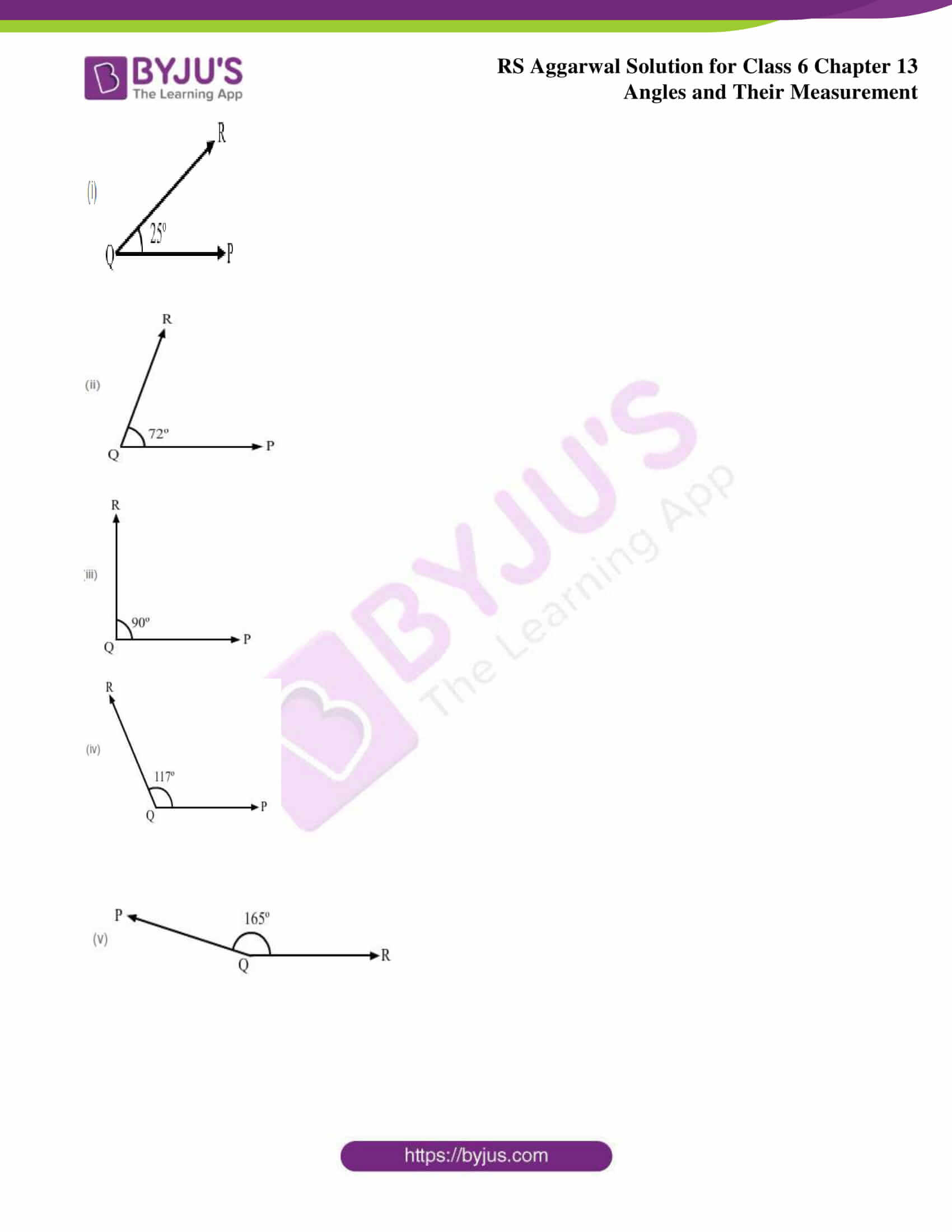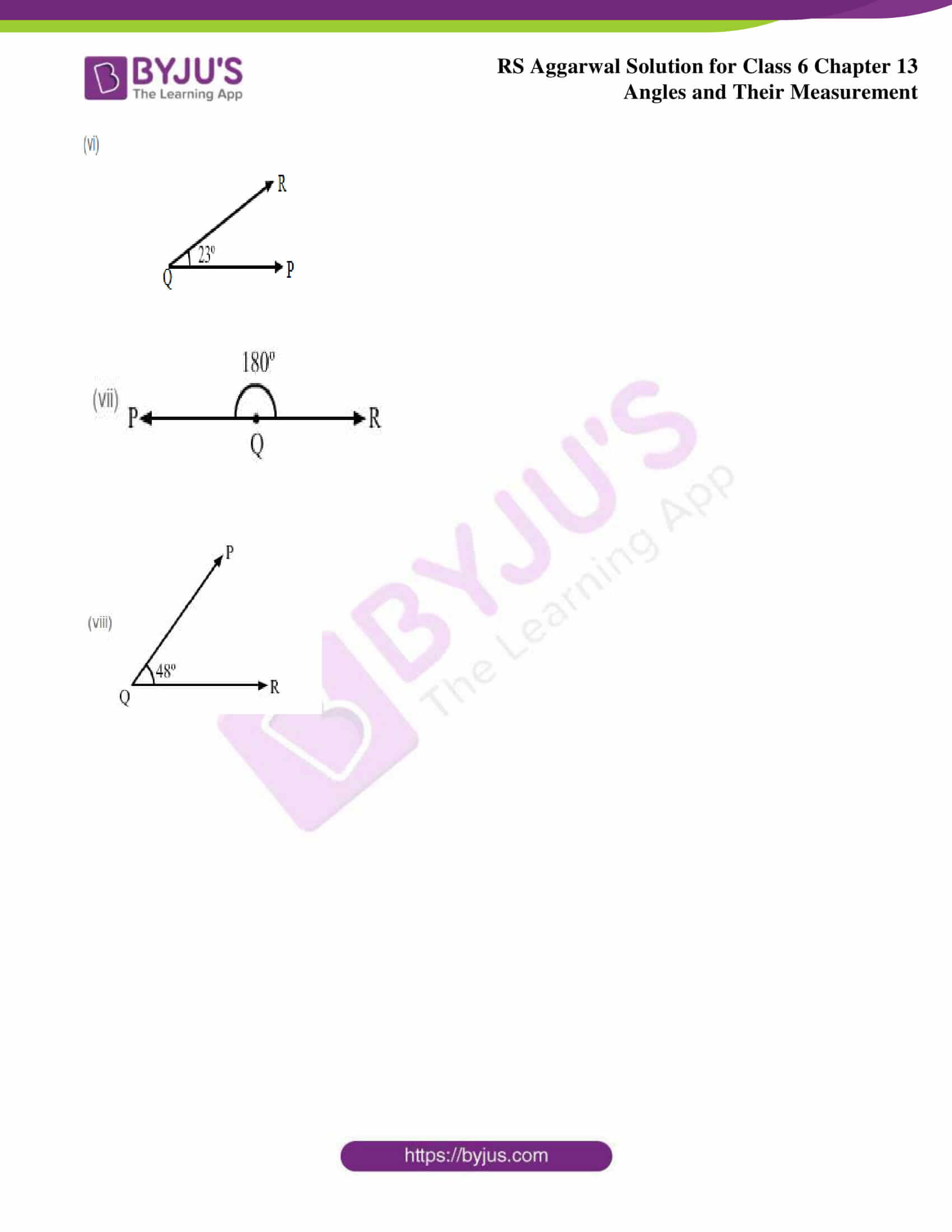### Access answers to Maths RS Aggarwal Solutions for Class 6 Chapter 13 Angles and their Measurement Exercise 13C

1. Measure each of the following angles with the help of a protractor and write the measure in degrees: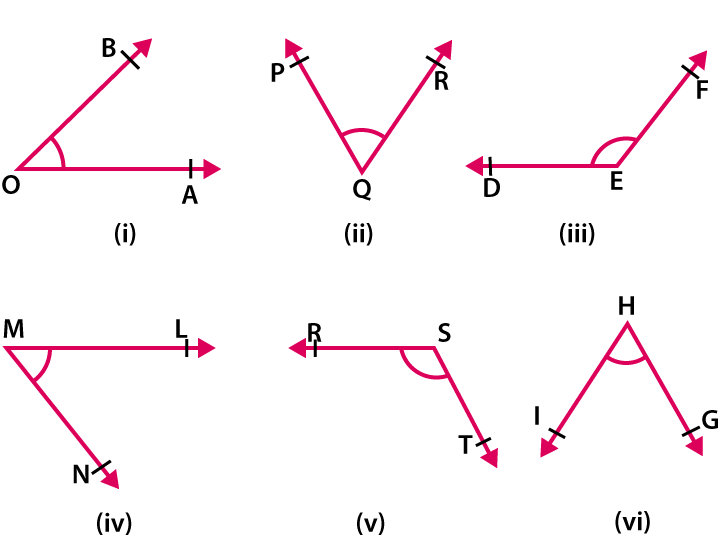Solution

(i) By measuring ∠BOA placing the protractor on one arm and measuring the angle through the other arm that coincides with the angle of the protractor is 450

(ii) By measuring ∠PQR placing the protractor on one arm and measuring the angle through the other arm that coincides with the angle of the protractor is 750

(iii) By measuring ∠DEF placing the protractor on one arm and measuring the angle through the other arm that coincides with the angle of the protractor is 1350

(iv) By measuring ∠LMN placing the protractor on one arm and measuring the angle through the other arm that coincides with the angle of the protractor is 550

(v) By measuring ∠RST placing the protractor on one arm and measuring the angle through the other arm that coincides with the angle of the protractor is 1350

(vi) By measuring ∠GHI placing the protractor on one arm and measuring the angle through the other arm that coincides with the angle of the protractor is 750

2. Construct each of the following angles with the help of a protractor:

(i) 250

(ii) 720

(iii) 900

(iv) 1170

(v) 1650

(vi) 230

(vii) 1800

(viii) 480

Solution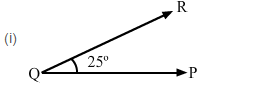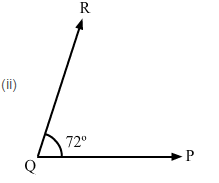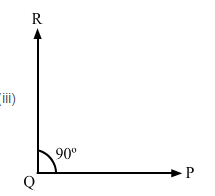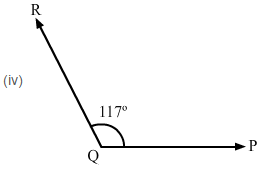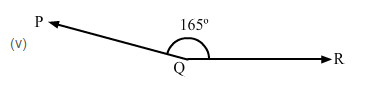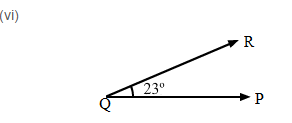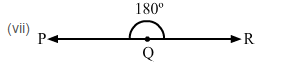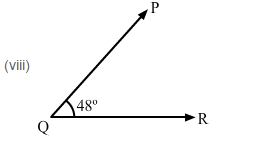### Access other exercises of RS Aggarwal Solutions for Class 6 Chapter 13 Angles and their Measurement

Exercise 13D Solutions